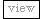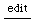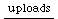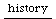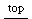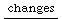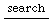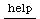## tetralemma - some logic

These algorithms create logical sentences in a certain random but consequential manner.
Julian Rohrhuber, 2006

"Neither either true or false nor both true and false."

Note that effectively (in terms of their extension) those sentences can be reduced to a simpler form using one of the basic logic operations. Nevertheless, what they express in their description (intension) is something else than their effective truth value. In Logic systems in which the tertium non datur (Law of the exluded middle) is not assumed, for example in intuitionistic logic, such expressions are not reducible to simpler expressions necessarily.

Here is a truth table as an example for a reduction:
```{|x,y| (((x and: y) xor: (not(x) and: not(y))) and: (not((x xor: y)) and: not((x and: y))))}

T	T	F
T	F	F
F	T	F
F	F	T

the function
{|x, y| not(x or: y) }
has the same output.
```

This scheme uses the form of the tetralemma (catuskoti) in indian logic as a basis and extrapolates it recursively. In the tetralemma "Everything is either true, or not true, or both true and not true, or neither true nor not true", there are four separate cases. I have combined the first two into one for formal reasons. (Note that Nagarjuna negates all four of them)

```// english

(
a = ["either % or %", "both % and %", "neither % nor %"];
x = {Ā|i, x=("true"), y=("false")| format(a[i], x, y) };
f = { #[0, 1, 2].scramble.keep(2) };
g = { |i=0, n=1, s1, s2|
var k, l;
#k, l = f.value;
if(n1) {
x.(
i,
g.(k, n-1, s1, s2),
g.(l, n-1, s1, s2)
)

}Ā{
x.(i, s1, s2)
}
};

z = {|n=1, s1="true", s2="false"|
var r = g.([0, 1, 2].choose, n, s1, s2);
r = r.toUpper;
quote(r ++ ".")
}
);

z.(1)
z.(2)
z.(3)

// or in numbers:

z.(1, 0, 1)
z.(2, 0, 1)
z.(3, 0, 1)

// if you don't think this kind of logic has its applications:

z.(1, "hungry", "tired");
z.(2, "hungry", "tired");
z.(3, "hungry", "tired");

// boolean logic formulation
a = ["(% xor: %)", "(% and: %)", "(not(%) and: not(%))"];

r = ("{|x,y| " ++ g.(1, 3, "x", "y") ++ "}").postln.interpret

r.(true, true)
r.(true, false)
r.(false, false)
r.(false, true)

// truth table:
(
var k = {|c|Āif(c) { "T" } { "F" } }.flop;
{: [x, y, r.(x, y)], x-[true, false], y-[true, false] }.do {|c| postf("%\t%\t%\n",*k.(c)) };
)

// sonification.
(
var n = 3; // number of levels
var j = -1;

g = { |i=0, n=1, s1, s2|
var k, l;
#k, l = f.value;
if(n>1) {
k = g.(k, n-1, s1, s2);
l = g.(l, n-1, s1, s2);

format("(u = %; Out.kr(%, u); u)", x.(i, k, l), j = j + 1)

}Ā{
format("(u = %; Out.kr(%, u); u)", x.(i, s1, s2), j = j + 1)
}
};
a = [
"{ m = %; l = %; (((l  1)*m) + ((m  1)* l)) >0 }.value",
"(%*%)",
"((%  1)*(%  1))"
];
r = ("{|x,y| var u, m, l; " ++ g.([0, 1, 2].choose, n, "x", "y") ++ " }").postln.interpret;
(
{
var a, b, x, amps, u;
a = LFPulse.kr(MouseX.kr(0.1, 100, 1), 0, 0.5);
b = LFPulse.kr(MouseY.kr(0.1, 100, 1), 0, 0.5);
r.(a, b);
amps = [a, b] ++ In.kr(0, j);
u = amps.collect { |a, i|
var freq = i.linexp(0, amps.size-1, 300, 6000);
a = a*AmpCompA.ir(freq);
SinOsc.ar(freq, 0, a)
};
Splay.ar(u)* (1/n)
}.play;
);
)

// german version
(
a = ["entweder % oder %", "sowohl % als auch %", "weder % noch %"];
x = {Ā|i, x=("wahr"), y=("falsch")| format(a[i], x, y) };
f = { #[0, 1, 2].scramble.keep(2) };
g = { |i=0, n=1, s1, s2|
var k, l;
#k, l = f.value;
if(n>1) {
x.(
i,
g.(k, n-1, s1, s2),
g.(l, n-1, s1, s2)
)

}Ā{
x.(i,*[s1, s2].scramble)
}
};
z = {|n=1, s1="wahr", s2="falsch"|
var r = g.([0, 1, 2].choose, n, s1, s2);
r = r.toUpper;
quote(r ++ ".")
}
)

z.(1)
z.(2)
z.(3)
```

### the buddhist tetralemma (catuskoti) extrapolation

Some Logical Aspects of Naagaarjuna's System
http://nyaya.darsana.org/topic56.html

``` "Everything is either true, or not true, or
both true and not true, or neither true nor
not true; that is the Buddhas teaching."
```

```(
a = ["either % or %", "both % and %", "neither % nor %"];
x = {Ā|i, x=("true"), y=("false")| format(a[i], x, y) };
f = { #[0, 1, 2].scramble.keep(2) };
g = { |i=0, n=1, s1, s2|
var k, l;
#k, l = f.value;
if(n>1) {
x.(
i,
g.(k, n-1, s1, s2),
g.(l, n-1, s1, s2)
)

}Ā{
x.(i, s1, s2)
}
};

z = {|n=1, s1="true", s2="false"|
var r = g.([0, 1, 2].choose, n, s1, s2);
r = r.toUpper;
quote(r ++ ".")
}
);

z.(1, "true", "not true")
z.(2)
z.(3)
```

### This was challenged by Nagarjuna:

 "For entity and negation of entity do not
occur within a unity." (7.30)(10)
 "For real and non-real, being mutually
contradictory, do not occur in the same locus."
(8.7)
Applications of the rule with narrower values
for the terms are common:
 "For birth and death do not occur at the
same time." (21.3)
 "Nirvaa.na cannot be both entity and
non-entity, (since) nirvaa.,na is unconditioned, and
entity and non-entity are conditioned." (25.13)
 "For the two do not occur within one
place, just as light and darkness do not."
(25.14)(11)
 "He would be non-eternal and eternal, and
The law of the excluded middle is twice invoked
explicitly:
 "Other than goer and non-goer, there is no
third one that goes." (2.8)(12)
 "Other than goer and non-goer, there is no
third one that stays." (2.15)
In other examples, "tertium non datur" is tacitly
assumed.
 "He who posits an entity becomes
entangled in eternalism and annihilism, since that
entity